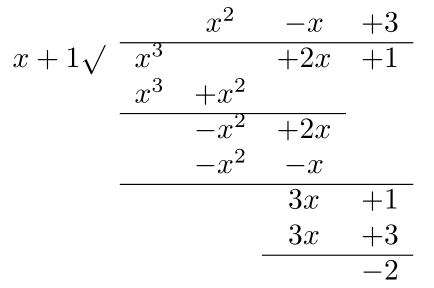## Random posts of math questions and solutions

### Polynomial Division### Polynomial Division

Number of replies: 0

Let $$f(x), p(x)$$ be two polynomials. Assume than the degree of $$f(x$$ is bigger than the degree of $$p(x)$$, then we can find a unique quotient (polynomial) $$q(x)$$ and a unique remainder polynomial $$r(x)$$, such that

$$f(x)=p(x)q(x)+r(x).$$

Question: If $$f(x)=x^3+2x+1, p(x)=x+1$$, what is $$q(x)$$ and $$r(x)$$?Thus, $$q(x)=x^2-x+3, r(x)=-2$$.